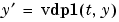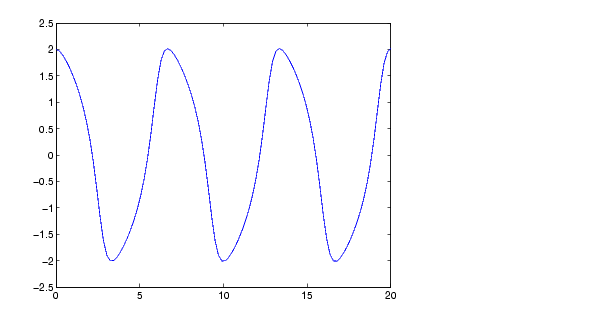MATLAB Function Referencedeval

Evaluate the solution of a differential equation

Syntax

• ```sxint = deval(sol,xint)
sxint = deval(xint,sol)
sxint = deval(sol,xint,idx)
sxint = deval(xint,sol,idx)
[sxint, spxint] = deval(...)
```

Description

```sxint = deval(sol,xint) and sxint = deval(xint,sol) ``` evaluate the solution of a differential equation problem. `sol` is a structure returned by one of these solvers:

• An initial value problem solver (`ode45`, `ode23`, `ode113`, `ode15s`, `ode23s`, `ode23t`, `ode23tb`, `ode15i`)
• The delay differential equations solver (`dde23`),
• The boundary value problem solver (`bvp4c`).

`xint` is a point or a vector of points at which you want the solution. The elements of `xint` must be in the interval `[sol.x(1),sol.x(end)]`. For each `i`, `sxint(:,i)` is the solution at `xint(i)`.

```sxint = deval(sol,xint,idx) and sxint = deval(xint,sol,idx) ``` evaluate as above but return only the solution components with indices listed in the vector `idx`.

`[sxint, spxint] = deval(...)` also returns `spxint`, the value of the first derivative of the polynomial interpolating the solution.

 Note    For multipoint boundary value problems, the solution obtained by `bvp4c` might be discontinuous at the interfaces. For an interface point `xc`, `deval` returns the average of the limits from the left and right of `xc`. To get the limit values, set the `xint` argument of `deval` to be slightly smaller or slightly larger than `xc`.

Example

This example solves the systemusing `ode45`, and evaluates and plots the first component of the solution at 100 points in the interval `[0,20]`.

• ```sol = ode45(@vdp1,[0 20],[2 0]);
x = linspace(0,20,100);
y = deval(sol,x,1);
plot(x,y);```

See Also

ODE solvers: `ode45`, `ode23`, `ode113`, `ode15s`, `ode23s`, `ode23t`, `ode23tb`, `ode15i`

DDE solver: `dde23`

BVP solver: `bvp4c`

© 1994-2005 The MathWorks, Inc.